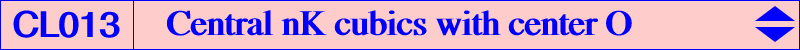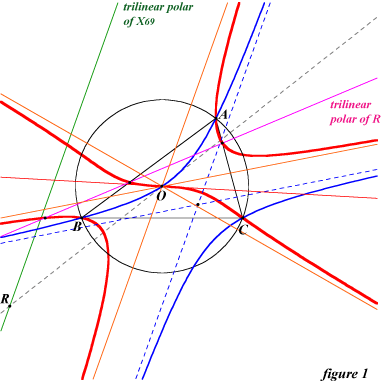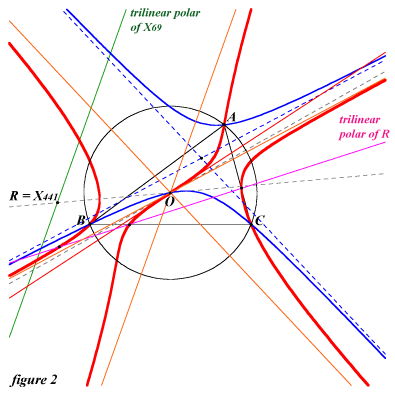All central nKs with center O = X(3) have their pole W on the trilinear polar of O and their root R on the trilinear polar of X(69). W is the isogonal conjugate of the tripole of a line L passing through H. The asymptotes of the cubic are : one (always real) which is perpendicular at O to L, two (not always real) which are parallel at O to those of the conic isogonal transform of L. For example, when the line is the Euler line, the cubic is K069 with three real points at infinity : X(523) and those of the Jerabek hyperbola. See a generalization in the page P-conical cubics. The figure 1 shows the cubic with pole X(684) and the circum-conic through O and X(76). The three points at infinity are X(511), X(525) and X(2799). The figure 2 shows the cubic with pole X(520), root X(441) and the circum-conic through O and G. The three points at infinity are X(525) and those of the conic.